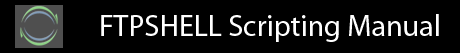### 1.5 Conditional execution

A conditional statement consists of the keyword if followed by the test condition and a statement block. The statement block is mandatory. An else keyword may also be used to specify an alternate condition.

String comparison operators are:

`eq    (equality)ne    (inequality)lt    (less than)le    (less than or equal to)gt    (greater than)ge    (greater than or equal to)`

Numeric comparison operators are:

`==    (equality)!=    (inequality)<     (less than)<=    (less than or equal to)>     (greater than)>=    (greater than or equal to)`

Arguments are automatically treated as strings or numbers based on the comparison operator that is used. Strings may also contain wildcards such as *.

The syntax is

 `if beginendif beginend else beginend`

Some examples are

 `if ~numvar < 5 beginendif ~strvar eq "*.txt" beginend else beginend`

Previous | Next